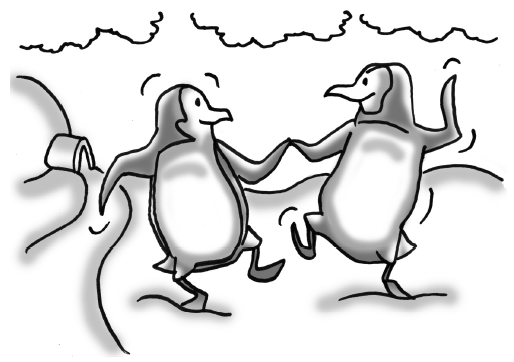### Home > CCG > Chapter 3 > Lesson 3.1.4 > Problem3-43

3-43.Frank and Alice are penguins. At birth, Frank’s beak was $1.95$ inches long, while Alice’s was $1.50$ inches long.

1. Frank’s beak grows by $0.25$ inches per year and Alice’s grows by $0.40$ inches per year. Write an equation to represent the length of each penguin’s beak in $x$ years.

Frank: $b=1.95+0.25y$ where $b=\text{beak length (inches)}$ and $y = \text{time (years)}$

Use the same type of equation for Alice.

2. How old will they be when their beaks are the same length?

What must be true about the two equations if their beaks are the same length?